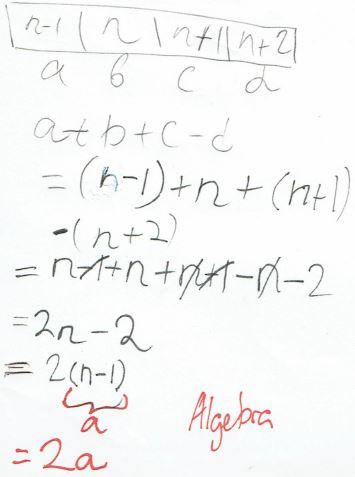#### You may also like### Network Trees

Explore some of the different types of network, and prove a result about network trees.### Always Two

Find all the triples of numbers a, b, c such that each one of them plus the product of the other two is always 2.### Symmetricality

Five equations and five unknowns. Is there an easy way to find the unknown values?

# Starting to Explore Four Consecutive Numbers

##### Age 11 to 16Challenge Level

1. Four consecutive numbers which sum to $130,$ and four consecutive numbers which sum to $-38$

Well done to Kaylie and Zoe and Alexis, Angus and Andrew and Aila from Frederick Irwin Anglican School in Australia, Charlie from Knutsford Academy and Adam_students from Torlands in England, and Sara, Theadora, Veesal and Lily A from Montpelier High School in the UK, who all found the four consecutive numbers which sum to $130.$ Kaylie and Zoe wrote:

130: 31 + 32 + 33 + 34
-38: -8 + (-9) + (-10) + (-11)

Sara also found four almost-consecutive numbers which sum to $130$:

-62 + 63 + 64 + 65 = 130
It took a few tries but we found 130 and they are almost consecutive numbers.

Thea from Montpelier High School showed how to use trial and error to find the answers. Click on each image to enlarge it:

Sireene and Aroosa from Montpelier High School and Ritheshkhanna from Wilson's Grammar School in England used number facts. Ritheshkhanna wrote:

First I made an educated guess by adding numbers 1-4  which is ten and with this new information I removed 10 from 130 giving me 120 left. Next I did 120 divided by four as there are four numbers and I got 30. Now I had the single digit consecutive numbers and the 2 digit numbers that accompany them. Finally, from this information I got the answer $a = 31, b = 32, c = 33, d = 34.$

Ayub from Portland Place in the UK, Kaia and Salma from Monteplier High School and Arjun, Nathan, Yunsung and Savith from Wilson's Grammar School used ideas about the mean. Arjun and Savith wrote:

a) $A+B+C+D=130$
As there are 4 numbers we should divide by 4 to find out what the number should be close to.
$130\div4=32.5$
Must be around that number
$31+32+33+34=130$

b) $A+B+C+D= -38$
As there are 4 numbers we should divide by 4 to find out what the number should be close to.
$38\div4=9.5$
Must be around that number
$-8+-9+-10+-11=-38$

Samuel from the UK, Aritro from St. Christopher's School in Bahrain, Anna and Elizabeth from Withington Girls’ School in England, Eva, Vladimir, Arman, Ivan, Ivan, Ekaterina and Sama from CIS Skolkovo in Russia, Yuvraj from DPS Rajnagar in India, Roger from Westfield Academy in the UK, Nikhil and Adhiraj T from Wilson's Grammar School, Edith from The British School in the Netherlands (Senior School Leidschenveen), Yuk-Chiu from Harrow School in the UK, Theekshi from NLCS in the UK and Rishik K used algebra, starting from the first number $a$. This is Edith's work for part (a):

Let the first term in the sequence be $a.$
Because the four terms are consecutive, each term is $1$ bigger than the number before it. As a result, the 2nd, 3rd and 4th terms in the sequence will be:
$a + 1, (a + 1) + 1, [(a + 1) + 1] + 1$
To simplify:
$a + 1, a + 2, a + 3$
As stated in the problem, the four terms sum to $130.$ To express it in an equation:
$a + (a + 1) + (a + 2) + (a + 3) = 130$
To simplify:
$a + a + 1 + a + 2 + a + 3 = 130$
$(a + a + a + a) + (1 + 2 + 3) = 130$
$4a + 6 = 130$
$4a = 124$
$a = 31$
As shown above, the first term of the sequence is $31.$ Now, we can plug it in the expressions and figure out the rest of the terms:
2nd term: $a + 1 = 31 + 1 = 32$
3rd term: $a + 2 = 31 + 2 = 33$
4th term: $a + 3 = 31 + 3 = 34$

The numbers are: $31, 32, 33$ and $34.$

CHECK:
The four terms should sum up to $130.$
$31 + 32 + 33 + 34 = 130$
As a result, the answer is correct.

This is Eva, Vladimir, Arman, Ivan, Ivan, Ekaterina and Sama's work for part (b):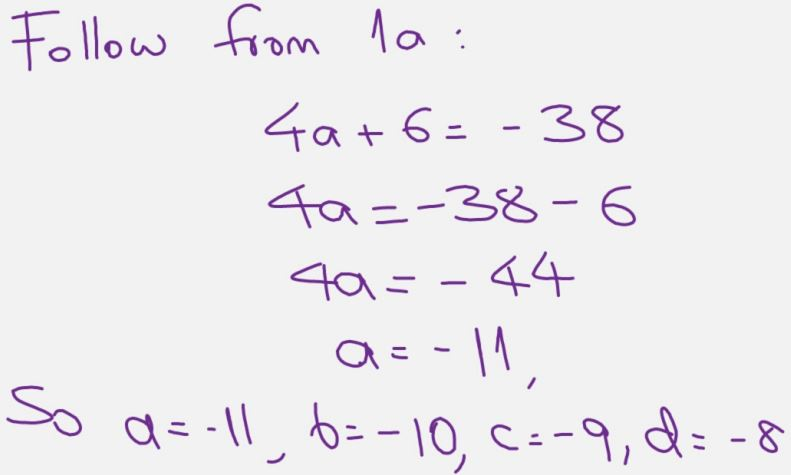Ci Hui Minh Ngoc Ong from Kelvin Grove State College (Brisbane) in Australia used a slightly different algebraic set up: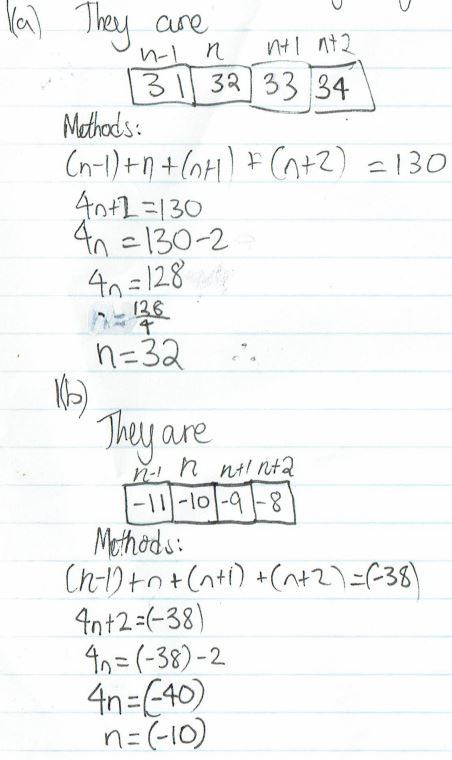2. The sum of the first three consecutive numbers is $10$ more than the fourth. What are the four numbers?

Sireene from Montpelier High School, Kaylie and Zoe, Alexis and Alia, Angus and Andrew, Theadora, Salma and Adam+students all found the numbers. Sireene wrote:

$5,6,7,8.$
I started at $7,8,9,10,$ then worked backwards until I got the right combinations.

Sophia from Montpelier High School, Kaia, Sireene, Yunsung, Veesal, Lily Arjun, Aroosa and Savith explained how they used trial and found patterns. This is Aroosa's work: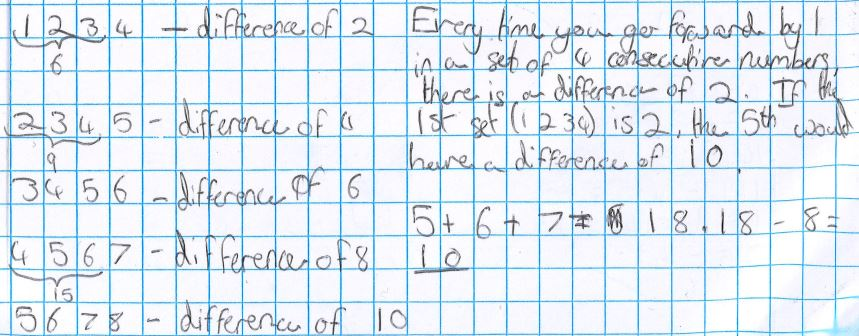Samuel, Aritro, Anna, Eva, Vladimir, Arman, Ivan, Ivan, Ekaterina and Sama, Yuvraj, Roger, Nihkil, Nathan, Kesav, Edith, Yuk-Chiu, Theekshi and Rishik K used algebra. This is Theekshi's work:

$a + b + c = d + 10$
$a + (a+1) + (a+2) = (a+3) + 10$
$3a + 3 = a + 13$
$2a = 10$
$a = 5$
$a = 5$  $b = 6$  $c = 7$  $d = 8$

Ci Hui Minh Ngoc Ong used the same method but different algebra again: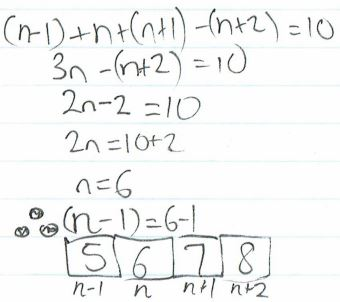3. What is $(a+d)-(b+c)$? Why?

Yunsung, Kaylie and Zoe, Alexis and Aila, Angus and Andrew, Sophia, Charlie, Veesal, Sireene, Aroosa, Salma, Arjun and Savith all found a rule. This is Angus and Andrew's work:

$(a+d) −(b+c)$ equals $0$ each time because $a+d$ is the equivalent to $b+c.$

e.g., $3+6$ is the equivalent to $4+5$ so taking each other will equal zero.

Another example is $(5432 + 5435) - (5433 + 5434) = 0$ because $5432 + 5435 = 10,867$ and $5433 + 5434 = 10,867$

Yuk-Chiu and Rishik K explained why the answer is always zero. This is Yuk-Chiu's explanation: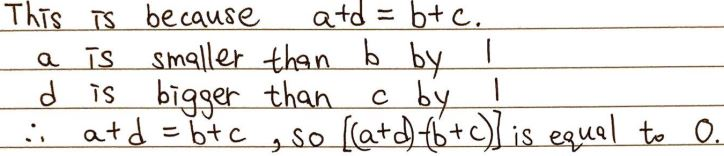Rishik wrote:

The smallest  and largest number can be balanced out by two middle numbers. $d-c=1.$ $a-b=-1.$

$(a+d) - (b+c) = a + d - b - c = (a-b) + (d-c) = -1+1=0$

Ayub used a similar idea and thought about the sum of the four numbers:

0, this is because $a+d = \tfrac12\text{sum},$  $b = a+1,c=d-1$ so $b+c=\tfrac12\text{sum}$ as well

Samuel, Aritro, Anna, Eva, Vladimir, Arman, Ivan, Ivan, Ekaterina and Sama, Elizabeth, Kaia, Yuvraj, Roger, Adam+students, Nikhil, Nathan, Adhiraj T, Edith, Yuk-Chiu, Theekshi and Rishik K used algebra starting from the first number. This is Roger's work:

Take the pair of four consecutive numbers: $5,6,7,8$ and $68,69,70,71.$

i. $5=n, 6=n+1,7=n+2$ and $8=n+3$
$(8+5)+(6+7)=13-13=0$

ii. $68=n, 69=n+1, 70=n+2, 71=n+3$
$(71+68)-(69+70)=139-139=0$

Explanation: $((n+3)+n)-((n+2)+(n+1))$
$=(2n+3)-(2n+3)=0$

Ci Hui Minh Ngoc Ong used the same method but different algebra again: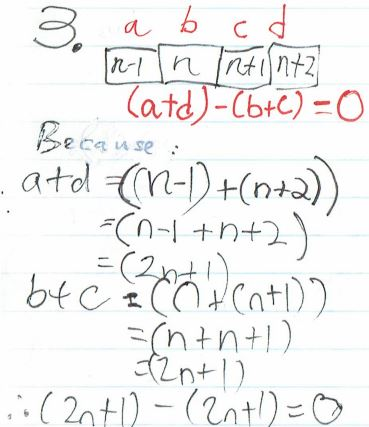4. Explore $a+b+c-d$.

Sophia, Angus and Andrew noticed some patterns. Sophia wrote: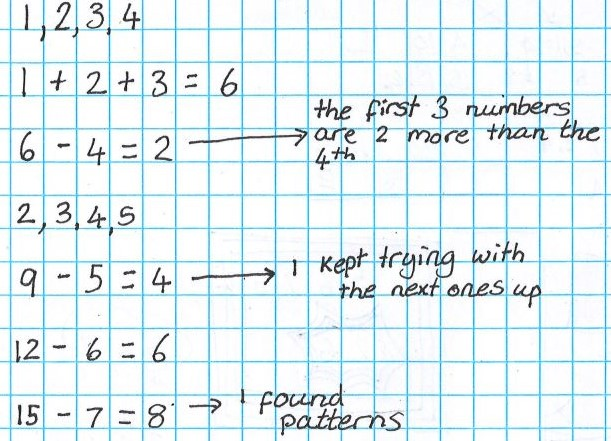Angus and Andrew described the patterns:

They are always even number answers. To add to that every time you go up a digit from $1+2+3-4$ they will go up by two’s.

Flo, Kaylie and Zoe, Alexis and Aila, Charlie, Arjun and Savith described the pattern in a different way. Alexis and Aila wrote:

$a+b+c-d=2a.$   $a+b+c-d$ is equal to $a$ times $2.$

Samuel, Aritro, Eva, Vladimir, Arman, Ivan, Ivan, Ekaterina and Sama, Elizabeth, Yuvraj, Roger, Nikhil, Adhiraj T, Edith, Yuk-Chiu, Theekshi and Rishik K used algebra to show that this is always true. This is Samuel's work: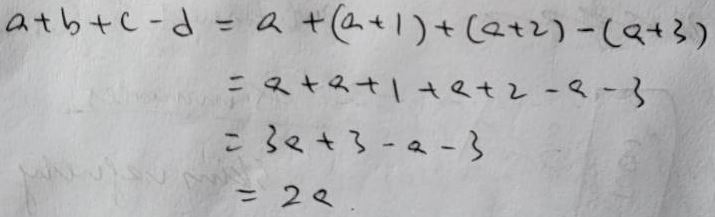Ci Hui Minh Ngoc Ong used the same method with different algebra: